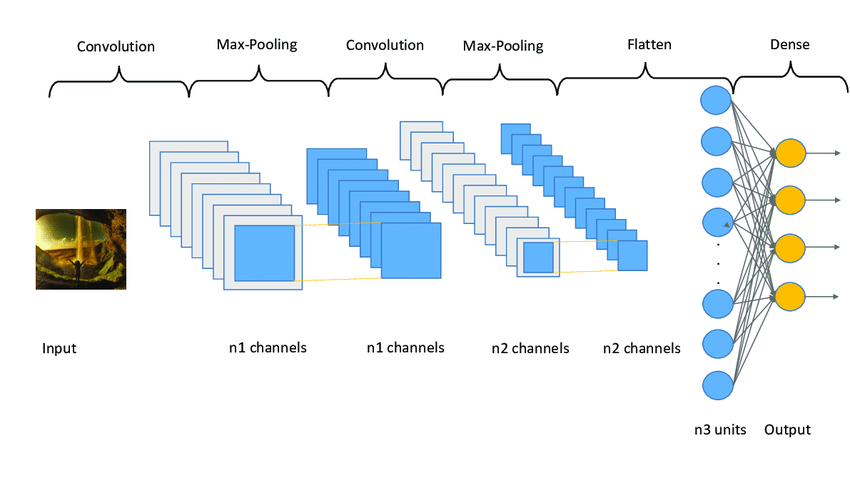Open in App
Not now

# Python Tensorflow – tf.keras.layers.Conv2D() Function

• Last Updated : 21 Mar, 2022

In this article, we shall look at the in-depth use of tf.keras.layers.Conv2D() in a python programming language.

## Convolution Neural Network: CNN

Computer Vision is changing the world by training machines with large data to imitate human vision. A Convolutional Neural Network (CNN) is a specific type of artificial neural network that uses perceptrons/computer graphs, a machine learning unit algorithm used to analyze data. This data mainly involves images. A 3D  vector dimension is passed through feature maps and then this is downsampled using the Pooling technique. The widely used Pooling technique to downsample the image feature maps is MaxPooling and MeanPooling.## Application Of CNN:

Convolution Neural Network is widely used in Computer Vision Technology, the main application includes:

• Object Detection
• Classification: Breast Cancer Prediction
• Semantic Segmentation
• Self-driving
• Probability Control

## CNN Implementation In Keras: tk.keras.layers.Conv2D()

#### Class Structure Of Conv2D:

tf.keras.layers.Conv2D(filters, kernel_size, strides=(1, 1), padding=”valid”, data_format=None, dilation_rate=(1, 1), groups=1, activation=None, use_bias=True, kernel_initializer=”glorot_uniform”, bias_initializer=”zeros”, kernel_regularizer=None, bias_regularizer=None, activity_regularizer=None, kernel_constraint=None, bias_constraint=None, **kwargs)

The commonly used arguments of tk.keras.layers.Conv2D() filters, kernel_size, strides, padding, activation.

## Convolution Neural Network Using Tensorflow:

Convolution Neural Network is a widely used Deep Learning algorithm. The main purpose of using CNN is to scale down the input shape. In the example below we take 4 dimension image pixels with a total number of 50 images data of 64 pixels. Since we know that an image is made of three colors i.e. RGB, thus the 4 value 3 denotes a color image.

On passing the input image pixel to Conv2D it scales down the input size.

Example:

## Python3

 `import` `tensorflow as tf``import` `tensorflow.keras as keras` `image_pixel ``=` `(``50``,``64``,``64``,``3``)``cnn_feature ``=` `tf.random.normal(image_pixel)``cnn_label ``=` `keras.layers.Conv2D(``2``, ``3``, activation``=``'relu'``,``                                ``input_shape``=``image_pixel[``1``:])(``  ``cnn_feature)``print``(cnn_label.shape)`

Output:

`(50, 62, 62, 2)`

By providing padding argument as same the input size shall remain the same.

## Python3

 `image_pixel ``=` `(``50``, ``64``, ``64``, ``3``)``cnn_feature ``=` `tf.random.normal(image_pixel)``cnn_label ``=` `keras.layers.Conv2D(``    ``2``, ``3``, activation``=``'relu'``,``  ``padding``=``"same"``,``  ``input_shape``=``image_pixel[``1``:])(cnn_feature)``print``(cnn_label.shape)`

Output:

`(50, 64, 64, 2)`

The pixel-sized is unchanged as we have provided padding to be the same.

## Implementing keras.layers.Conv2D() Model:

Putting everything learned so far into practice. First, we create a Keras Sequential Model and create a Convolution layer with 32 feature maps at size (3,3). Relu is the activation is used and later we downsample the data by using the MaxPooling technique. We further scale down the image by passing it through the second Convolution layer with 64 feature maps. This process is called Feature Extraction. Once feature extraction is done, we can flatten the data into a single vector and feed them to hidden dense layers. The softmax activation is used at the output layer to make sure these outputs are of categorical data type which is helpful for Image Classification.

## Python3

 `import` `tensorflow.keras as keras`  `def` `build_model():``    ``model ``=` `keras.Sequential(``        ``[``            ``# first convolution layer``            ``keras.layers.Conv2D(``32``, (``3``, ``3``), activation``=``"relu"``,``                                ``input_shape``=``(``32``, ``32``, ``3``)),``            ``keras.layers.MaxPooling2D((``2``, ``2``), strides``=``2``),` `            ``# second convolution layer``            ``keras.layers.Conv2D(``64``, (``3``, ``3``), activation``=``"relu"``),``            ``keras.layers.MaxPooling2D((``2``, ``2``), strides``=``2``),` `            ``# fully connected classification``            ``# single vector``            ``keras.layers.Flatten(),``          ` `            ``# hidden layer and output layer``            ``keras.layers.Dense(``1024``, activation``=``"relu"``),``            ``keras.layers.Dense(``10``, activation``=``"softmax"``)``        ``])``    ``return` `model`

Output:

`<keras.engine.sequential.Sequential object at 0x7f436e8bc2b0>`

My Personal Notes arrow_drop_up